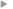## Scaling of Differential Equations

#### Langtangen, Hans Petter ; Pedersen Geir K.

Editeur
Springer International Publishing
Année de publication
2016
ISBN
978-3-319-32726-6
Résumé
The book serves both as a reference for various scaled models with corresponding dimensionless numbers, and as a resource for learning the art of scaling. A special feature of the book is the emphasis on how to create software for scaled models, based on existing software for unscaled models. Scaling (or non-dimensionalization) is a mathematical technique that greatly simplifies the setting of input parameters in numerical simulations. Moreover, scaling enhances the understanding of how different physical processes interact in a differential equation model. Compared to the existing literature, where the topic of scaling is frequently encountered, but very often in only a brief and shallow setting, the present book gives much more thorough explanations of how to reason about finding the right scales. This process is highly problem dependent, and therefore the book features a lot of worked examples, from very simple ODEs to systems of PDEs, especially from fluid mechanics. The text is easily accessible and example-driven. The first part on ODEs fits even a lower undergraduate level, while the most advanced multiphysics fluid mechanics examples target the graduate level. The scientific literature is full of scaled models, but in most of the cases, the scales are just stated without thorough mathematical reasoning. This book explains how the scales are found mathematically. This book will be a valuable read for anyone doing numerical simulations based on ordinary or partial differential equations.
Texte intégralAccès à la notice sur le site du portail documentaire de Météo-FranceListe complète des notices publiques